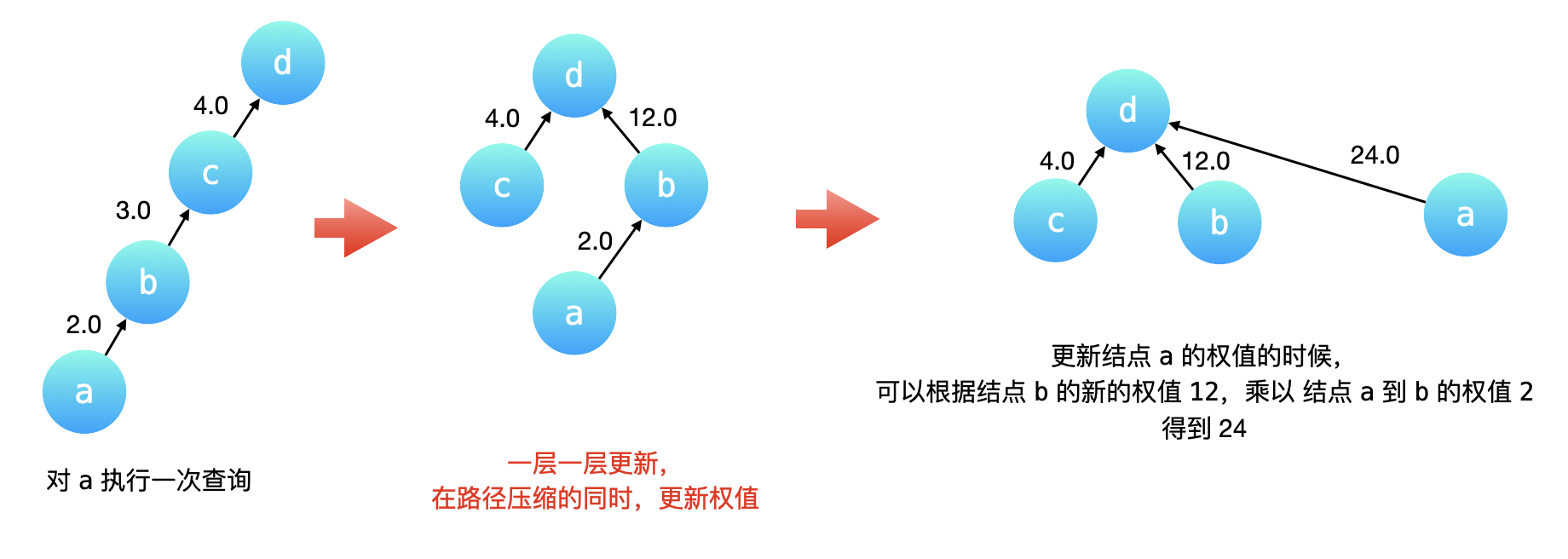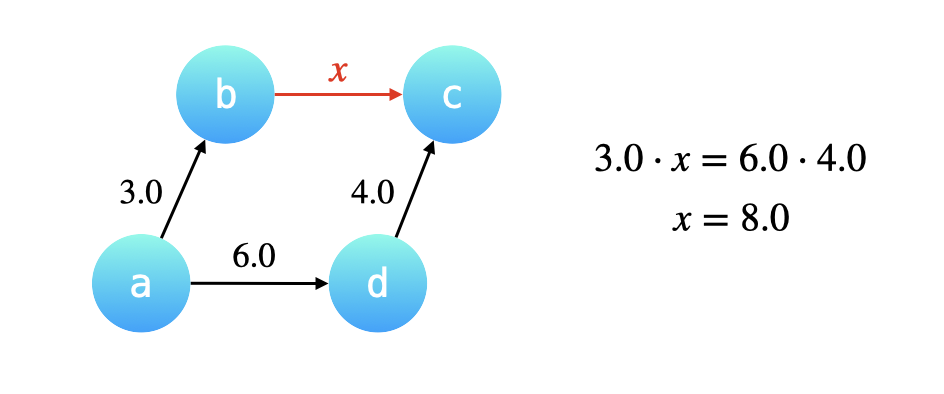# 并查集

• 查询（Find）：查询某个元素属于哪个集合，通常是返回集合内的一个“代表元素”。这个操作是为了判断两个元素是否在同一个集合之中。（并查集只知道两个顶点之间是否有一条路径连接，而不知道如何连接）
• 合并（Union）：将两个集合合并为一个。
• 添加：添加一个新集合，其中有一个新元素。添加操作不如查询和合并操作重要，常常被忽略。

“并查集”可以用来指代任何支持上述操作的数据结构，但是一般来说，“并查集”特指其中最常见的一种实现：不交集森林（Disjoint-set forest）。经过优化的不交集森林有线性的空间复杂度（$$O(n)$$$$n$$ 为元素数目，下同），以及接近常数的单次操作平均时间复杂度（$$O(\alpha(n))$$$$\alpha$$反阿克曼函数），是效率最高的常见数据结构之一。

### 实现¶

1. quick-find 基于 id
• 相同的集合分配一个唯一的标识，称为 id；
• 初始化：所有元素的 id 都不同，表示每个元素单独属于一个集合；
• 不同元素如果 id 相同，表示属于同一个集合；
• 查询时可直接得知属于哪个集合；
• 合并集合时，将被合并的所有元素的 id 设置为目标集合的 id。
2. quick-union 基于 parent
• 只记录节点（元素）属于哪一个父亲节点，这样设计「并查集」的思想也叫「代表元」法；
• 查询时需要不断向上查找父亲节点，直到找到根节点，如果根节点相同，表示属于同一个集合；
• 合并时只需要将被合并元素的根节点的父亲节点设置为目标集合的根节点；
• 从总体上来说，这种结构和树结构相似，只不过前者从下往上访问，而后者从上往下访问。

#### 代表元法¶

##### A. 按秩合并¶

• 按 size 合并，用于需要维护每个连通分量结点个数的时候；
• 按 rank 合并，绝大多数时候。这里的 rank 指的是树的（大致）高度。

##### 代码实现¶
class Dsu {
public:
Dsu(size_t n): parents(n), sizes(n, 1) {
iota(parents.begin(), parents.end(), 0);
}
void unite(int a, int b) {
if (a == b) return;
int rootA = find(a);
int rootB = find(b);
if (rootA == rootB) return;
if (sizes[rootA] < sizes[rootB]) {
parents[rootA] = rootB;
sizes[rootB] += sizes[rootA];
} else {
parents[rootB] = rootA;
sizes[rootA] += sizes[rootB];
}
}
int find(int a) {
if (parents[a] != a) {
parents[a] = find(parents[a]);
}
return parents[a];
}
private:
vector<int> parents;
vector<int> sizes;
};


#### 带权并查集¶

##### 在「查询」操作的「路径压缩」优化中维护权值变化¶

• c 的父亲结点已经是根结点了，它的权值不用更改；
• b 的父亲结点要修改成根结点，它的权值就是从当前结点到根结点经过的所有有向边的权值的乘积，因此是 $$3.0$$ 乘以 $$4.0$$ 也就是 $$12.0$$
• a 的父亲结点要修改成根结点，它的权值就是依然是从当前结点到根结点经过的所有有向边的权值的乘积，但是我们没有必要把这三条有向边的权值乘起来，这是因为 b 到 c，c 到 d 这两条有向边的权值的乘积，我们在把 b 指向 d 的时候已经计算出来了。因此，a 到根结点的权值就等于 b 到根结点 d 的新的权值乘以 a 到 b 的原来的有向边的权值。##### 如何在「合并」操作中维护权值的变化¶

「合并」操作基于这样一个 很重要的前提：我们将要合并的两棵树的高度最多为 $$2$$，换句话说两棵树都必需是「路径压缩」以后的效果，两棵树的叶子结点到根结点最多只需要经过一条有向边。##### 代码实现¶
class WeightedDsu {
public:
WeightedDsu(size_t n): parents(n), sizes(n, 1), weights(n, 1.0) {
iota(parents.begin(), parents.end(), 0);
}
void unite(int a, int b, double value) {
if (a == b) return;
int rootA = find(a);
int rootB = find(b);
if (rootA == rootB) return;
if (sizes[rootA] < sizes[rootB]) {
parents[rootA] = rootB;
weights[rootA] = weights[b] * value / weights[a];
sizes[rootB] += sizes[rootA];
} else {
parents[rootB] = rootA;
weights[rootB] = weights[a] / (weights[b] * value);
sizes[rootA] += sizes[rootB];
}
}
int find(int a) {
if (parents[a] != a) {
int origin = parents[a];
parents[a] = find(parents[a]);
weights[a] *= weights[origin];
}
return parents[a];
}
double weight(int a) {
return weights[a];
}
private:
vector<int> parents;
vector<int> sizes;
vector<double> weights;
};


#### 可变长度版¶

parentssizes 都使用 unordered_map 类型，在调用 find 方法时，动态添加新元素。

class DynamicDsu {
public:
DynamicDsu() {
count = 0;
}
void unite(int a, int b) {
if (a == b) return;
int rootA = find(a);
int rootB = find(b);
if (rootA == rootB) return;
if (sizes[rootA] < sizes[rootB]) {
parents[rootA] = rootB;
sizes[rootB] += sizes[rootA];
} else {
parents[rootB] = rootA;
sizes[rootA] += sizes[rootB];
}
count--;
}
int find(int a) {
if (parents.count(a) == 0) {
parents[a] = a;
sizes[a] = 1;
count++;
return a;
}
if (parents[a] != a) {
parents[a] = find(parents[a]);
}
return parents[a];
}
int findOrCreate(int a) {
if (parents[a] != a) {
parents[a] = find(parents[a]);
}
return parents[a];
}
int getCount() {
return count;
}
private:
int count;
unordered_map<int, int> parents;
unordered_map<int, int> sizes;
};